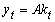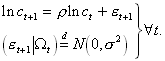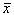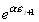John Hassler

March 31, 1995

EXAM
Macroeconomics I
5 points

Choose 4 out of the following 5. 1 page per question should be enough for your answers. Worth 10 points each.

1. Evaluate the following statement. "A temporarily high government spending, like a war, should be financed by temporarily high taxes."

2. Is the following statement true, false or uncertain? "Humans do not live for ever. The probability of dying implies that the Ricardian Equivalence theorem cannot hold."

3. Is the following statement true, false or uncertain? "The price of a risky asset is a random walk with drift, so its return is unpredictable."

4. Is the following statement true, false or uncertain? "Under full liquidity constraints the marginal propensity to consume out of current income is generally at least as large as under no liquidity constraints."

5. Is the following statement true, false or uncertain? "Fluctuations in labor input in a RBC model, not caused by real wage fluctuations, should be negatively correlated with consumption."

II Problems

Choose 1 out of the following 2. Provide a strict formal argument. Worth 25 points.

7. Consider a continuous-time model of a closed economy without population growth or technical progress. Production per capita, at date t, is given by7.1

where k denotes capital per capita and A is a productivity parameter. Capital depreciates at the rate dt, where dt is a convex function of aggregate capital Kt:with BK, BKK >0.

Consumers save in the form of capital; the representative consumer chooses an optimal consumption plan, so as to maximize7.2

where c is consumption per capita and r is the rate of time preference.

a) What will the representative firm be paying to rent capital from consumers in equilibrium at a competitive capital market, where each firm takes aggregate capital as given?

b) Write down the consumer’s budget constraint and characterize the solution to her intertemporal maximization problem.

c) Consider the full competitive market equilibrium in this economy; normalize the constant labor force to unity such that kt=Kt at all t. Characterize the equilibrium dynamics of ct and kt, both algebraically and in a common diagram! Does this economy converge to a steady state from any initial capital stock k0?

d) Is the private market equilibrium socially efficient from the viewpoint of a social planner that seeks to maximize the representative consumer’s utility function ? Explain why or why not, by analyzing the solution to for c and k in the social planner’s problem!

8. Consider an economy where the log of per capita consumption follows an AR(1) process with i.i.d. normal innovations,8.1

where the last line of the previous equation says that the distribution of et+1 conditional on information known in t equals in distribution a normal with variance s2. Now assume that all agents are identical and maximize8.2

a) What is the mean, variance and distribution ofand?

b) Recall that if x is normal with meanand variance s2 then the expected value. Use this to show that8.3

We are now opening markets for a few different assets in this economy. We make sure that the net supply of each asset is zero. Your task is to calculate the prices of these assets.

c) Is the net supply assumption important? Why or why not?

d) Calculate the price of a bond that returns 1 SEK the next period for sure. Explain in a few words how it depends on a, b, s, r and ct!

e) Let pt be the price calculated under d). Define the riskless rate of return as -lnpt. What is the time average of the riskless rate of return. Explain in a few words how it depends on a, b, s, r and ct!

f) Calculate the price of the following stochastic asset. The period after the asset is bought a dice is thrown (en tärning kastas). The holder of the asset receives the same number of SEK as the number of eyes the dice shows. Explain your results!

g) Calculate the price of a stochastic asset that paysif bought at t. Does this asset give a higher or lower return than the previous assets? Explain your results!

III Essay

Choose 1 out of the following 2. Emphasize discussion rather than formalism. Worth 25 points.

9. Growth Differences

Describe the stylized facts regarding differences across countries and time in the rate of growth in GDP per capita! Discuss both the methodology and the most important result in the empirical literature that seeks to explain these differences! What are the strong points and weak points of this work, in your view?

10. Asset Pricing Models

Discuss the empirical success and failures of the standard and consumption based CAPM? Indicate possible explanations to the problems you mention.

A pass will require 60 points (out of 100) and a pass with distinction 80 points.

Good Luck!